Courses

# Magnetics (Part - 1) - Physics, Solution by DC Pandey NEET Notes | EduRev

## JEE: Magnetics (Part - 1) - Physics, Solution by DC Pandey NEET Notes | EduRev

The document Magnetics (Part - 1) - Physics, Solution by DC Pandey NEET Notes | EduRev is a part of the JEE Course DC Pandey Solutions for NEET Physics.
All you need of JEE at this link: JEE

Introductory Exercise 23.1

Q.1. Write the dimensions of E/B. Here, E is the electric field and B the magnetic field.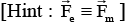Sol. qE = Bqv sin θ
[E/B] = [v] = [LT-1]

Q.2. In the relation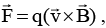which pairs are always perpendicular to each other.

Sol. From the property of cross product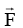is always perpendicular to both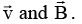Q.3. If a beam o f electrons travels in a straight line in a certain region. Can we say there is no magnet ic field?

Sol.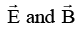both may be present and may be possible that,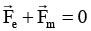Q.4. A charge q = -4μC has an instantaneous velocity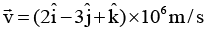in a uniform magnetic field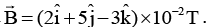What is the force on the charge?

Sol.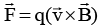Here, q has to be substituted with sign.

Introductory Exercise 23.2

Q.1. Can a charged particle be accelerated by a magnetic field. Can its speed be increased?

Sol Magnetic force may be non-zero. Hence acceleration due to magnetic force may be non-zero. Magnetic force is always perpendicular to velocity. Hence its power is always zero or work done by magnetic force is always zero. Hence it can be change the speed of charged particle.

Q.2. An electron beam projected along positive x-axis deflects along the positive y-axis. If this deflection is caused by a magnetic field, what is the direction of the field?

Sol: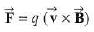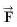is along position y -direction, q is negative and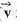is along positive x-direction. Therefore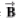should be along positive z-direction.

Q.3. An electron and a proton are projected with same velocity perpendicular to a magnetic field,
(a) Which particle will describe the smaller circle?
(b) Which particle will have greater frequency?

Sol.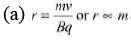as other factors are same.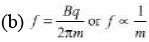Q.4. In a region of space a uniform magnetic field 6 is along positive x-axis. Electrons are emitted from the origin with speed v at different angles. Show that the paraxial electrons are refocused on the x-axis at a distance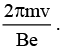Here, m is the mass of electron and e the charge on it.

Sol.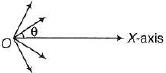Electrons touches the x-axis again after every pitch. Therefore the asked distance is d = p = v11T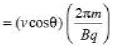For paraxial electrons θ ≈ 0° and q = e
∴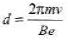Q.5. A particle of mass m and charge q is projected into a region having a perpendicular magnetic field 6. Find the angle of deviation of the particle as it comes out of the magnetic field if the width of the region is,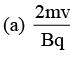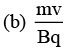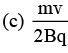Sol.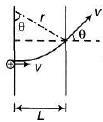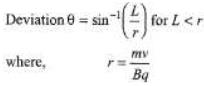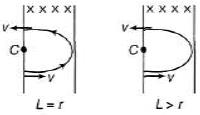and θ = π if L ≤ r

Q.6. An electron is accelerated through a PD of 100 V and then enters a region where it is moving perpendicular to a magnetic field S = 0.2 T. Find the radius of the circular path. Repeat this problem for a proton.

Sol.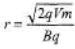Q.7. A proton, a deutron and an a-particle have equal kinetic energies. Compare the radii of their paths when a normal magnetic field is applied.

Sol.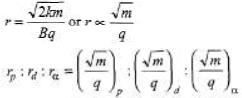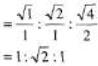The document Magnetics (Part - 1) - Physics, Solution by DC Pandey NEET Notes | EduRev is a part of the JEE Course DC Pandey Solutions for NEET Physics.
All you need of JEE at this link: JEEUse Code STAYHOME200 and get INR 200 additional OFF Use Coupon Code
All Tests, Videos & Notes of JEE: JEE

### Up next### Top Courses for JEE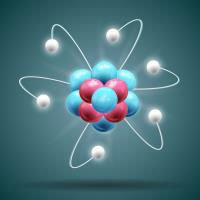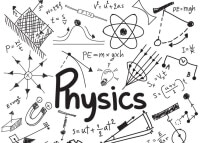## DC Pandey Solutions for NEET Physics

132 docs

### Up next### Top Courses for JEETrack your progress, build streaks, highlight & save important lessons and more!

,

,

,

,

,

,

,

,

,

,

,

,

,

,

,

,

,

,

,

,

,

,

,

,

;